# Hydration Features of Composite Binding Material with High-Dose Copper Tailings

Hydration Features of Composite Binding Material with High-Dose Copper Tailings
School of Urban Construction and Transportation, Hefei University, Hefei 230601, China

School of Electronic Science & Applied Physics, Hefei University of Technoogy, Hefei 230601, China

Corresponding Author Email:
2020213561@mail.hfut.edu.cn
Page:
17-24
|
DOI:
https://doi.org/10.18280/acsm.450103
28 November 2020
|
Accepted:
5 January 2021
|
Published:
28 February 2021
| Citation

OPEN ACCESS

Abstract:

Based on Krstulovic-Dabic model, this paper modifies the center particle hydration model according to the features of copper tailings, and then simulates the hydration process of cement-based composite binding system mixed with copper tailings. The hydration exothermic features were analyzed under different dosages, and different temperatures. Then, the simulated parameter values of the hydration process were compared with those measured in experiments. The results show that: the mixing of copper tailings powder can reduce the hydration heat release and slow down hydration rate of the composite binding material, and improve the thermal performance of the concrete; the prepared copper tailings powder is active enough to replace fly ash and ground-granulated blast furnace slag (GGBS) as cement admixtures; When the dosage surpasses 40%, the copper tailings can be applied to mass concrete projects.

Keywords:

high-dose copper tailings, composite binding material, hydration kinetics, hydration rate, hydration heat

1. Introduction

In cement production, the mixing of fly ash and blast furnance slag can increase the yield and variety of products and improve cement performance. However, traditional active admixtures like fly ash need to be partial replaced, in response to the optimization of energy structure, the rise of new energy, and the pursuit of energy saving and waste reuse. It is necessary to lower the production cost of cement using cheap and abundant mineral admixtures, and to produce “green cement” through full use of industrial waste. Copper tailings is a high-yield industrial waste with a small particle size. It is discharged in copper smelting after the extraction of useful metals like copper and gold.

Many scholars have explored the addition of mineral components as cement admixtures. Based on the characterization method of Ca (OH)2 content, Li et al.  studied the role of fly ash in composition binding system, and suggested that a high dosage of fly ash boosts cement hydration. Han et al.  examined the hydration properties of the slag-cement composite binding system under low temperature, and discovered that different low temperature conditions have different effects on the hydration of the system.

In conventional building structures, the hydration of cement creates a temperature gradient in the concrete. Then, the stress induced by the temperature difference will result in cracking, which in turn threatens the mechanical properties and durability of the structure . If a concrete structure is constructed in winter, the construction period will be extended, because the hydration is slowed down in the ultracold environment; in this case, the hydration heat helps to speed up the hydration reaction. For mass concrete, the cracking in the construction phase can be forecasted and mitigated effectively by studying the exothermic mechanism and cooling measures for the hydration of binding material . In actual construction, it is difficult to control the cracks, due to the heat release of cement hydration, as well as the interaction between temperature and stress fields. The effective crack control requires accurate calculation, analysis, and simulation of the temperature and stress of cement-based concrete .

Zhang  pointed out that adding fly ash or mineral powder can reduce the hydration heat and delay the hydration rate of binding material. Xie  found that the hydration heat of binding material can be very effectively lowered, when the dosage of fly ash-mineral powder surpasses 30%.

The relevant literature, such as those mentioned above, has not tackled the application of copper tailings as cement admixture. Taking copper tailings as cement admixture, this paper focuses on analyzing the rate and exothermic features of cement hydration under the effect of copper tailings. Based on Krstulovic-Dabic model, the authors proposed an improved hydration model for the composite binding material of copper tailings-cement. The research provides the theoretical basis for applying copper tailings and similar industrial waste (e.g., quartz tailings, iron tailings, and steel slags) as cement admixtures.

This paper will be organized as follows. In Section 2, the relationships of parameters such as hydration heat, hydration time, hydration degree, and particle size are created based on Krstulovic-Dabic model. In Section 3, Proposes hydration model of copper tailings-cement composite binding material. In Section 4, through experiments, the hydration rate and hydration heat of the composite binding material are measured. In Section 5, calculated and experimental values are compared and analyzed in detail. In Section 6, the conclusions are given.

2. Hydration Kinetics Model for Composite Binding Material

Krstulovic and Dabic  is a mainstream model for the hydration kinetics of cement-based materials. The model divides the hydraiton reaction into 3 basic phases: nucleation and crystal growth (NG), interactions at phase boundaries (I), and diffusion (D). On this basis, researchers have mainly studied the hydration kinetics of conventional cement admixtures like fly ash, ground-granulated blast furnace slag (GGBS), and silica fume.

According to Krstulovic-Dabic model, the kinetic equations between hydration degree (α) and reaction time (t) of the three phases and the corresponding differential equations can be expressed as:

Phase NG:

$K_{N G}\left(t-t_{0}\right)=[-\ln (1-\alpha)]^{\frac{1}{n}}$     (1)

$\frac{d \alpha}{d t}=K_{N G} n(1-\alpha)[-\ln (1-\alpha)]^{1-\frac{1}{n}}$     (2)

Phase I:

$K_{I}\left(t-t_{0}\right)=1-(1-\alpha)^{\frac{1}{3}}q$     (3)

$\frac{d \alpha}{d t}=3 K_{I}(1-\alpha)^{\frac{2}{3}}$     (4)

Phase D:

$K_{D}\left(t-t_{0}\right)=\left[1-(1-\alpha)^{\frac{1}{3}}\right]^{2}$     (5)

$\frac{d \alpha}{d t}=\frac{3}{2} K_{D}(1-\alpha)^{\frac{2}{3}}\left[1-(1-\alpha)^{\frac{1}{3}}\right]^{-1}$     (6)

where, KNG, KI, and KD are the hydration rate constants of the three phases, respectively.

Then, the authors further established the relationship between the hydration process and heat release of the binding material. The heat release of hydration can be expressed as a function of the hydration degree α :

$Q_{t}=\alpha \cdot Q_{T}$     (7)

Thus,

$\frac{d \alpha}{d t}=\frac{d Q}{d t} \frac{1}{Q_{T}}$     (8)

where, α is the hydration degree of cement; QT is the total heat release after the binding material is fully hydrated.

The total heat release of Portland cement can be determined by the Knudsen equation . The relationship between heat release and hydration time of cement can be described as:

$Q_{t}=\frac{Q_{I}\left(t-t_{0}\right)}{t+t_{h}-t_{0}}$     (9)

where, th is the time for the hydration to release half of the total heat releasable; t0 is the start time of hydration after the induction period of the cement.

The hydration degree of cement can be described as the ratio of cement heat release to the final total heat release of cement. Suppose the mineral components are uniformly distributed in cement particles, and the heat release of cement particles is proportional to the particle size . Then, the hydration degree α of cement particles with initial radius of R0 can be expressed as:

$\alpha=\frac{Q}{Q_{T}}=1-\frac{R^{3}(t)}{R_{0}^{3}}$     (10)

where, Q is the heat release of cement particles after being hydrated for time t; QT is the final total heat release of cement particles; R(t) is the radius of cement particles with initial radius of R0 at time t.

Substituting formula (10) into the Krstulovic-Dabic cement hydration formula, the variation of cement particle radius per unit time of hydration can be obtained as:

$\frac{d R_{N G}}{d t}=\frac{1}{3} n K_{N G} R(t)\left[-3 \ln \left(\frac{R(t)}{R_{0}}\right)\right]^{\frac{1}{n}}$     (11)

$\frac{d R_{I}}{d t}=K_{I} R_{0}$     (12)

$\frac{d R_{D}}{d t}=\frac{K_{D} R_{0}^{2}}{2\left[R_{0}-R(t)\right]}$     (13)

where, dRNG, dRI, and dRD are the radius reduction of cement particles with initial radius of R0 in the three phases of hydration, respectively; n is a constant related to the mineral composition of cement.

In this way, the expressions for the relationship among the following factors were derived from the Krstulovic-Dabic model: hydration heat, hydration time, hydration degree, and cement particle size.

3. Hydration Model for Composite Binding Material of Copper Tailings-Cement

3.1 Center particle hydration model for pure cement

So far, there is no research that explore or verify the composite binding material of copper tailings based on Krstulovic-Dabic model. For comparison, the center particle hydration model porposed by Tomosawa  was introduced. Taking cement as the only binding material, this model assumes that the cement particles are immersed in an infinite water environment, and neglects the impact of water cement ratio on the hydration process. On this basis, Park et al.  put forward a more practical hydration kinetics equation for pure cement, in view of mineral contents in cement, water cement ratio, cement particle diameter, and curing temperature, etc.:

$\begin{array}{c} \frac{d \alpha}{d t}=\frac{3 C_{w-\text { free }}}{r_{0} \rho_{c}\left(w / c+w_{a g}\right)}\left[\frac{D_{e}-k_{d} r_{0}}{k_{d} D_{e}}+\frac{r_{0}(1-\alpha)^{-\frac{1}{3}}}{D_{e}}\right. \\ \left.+\frac{(1-\alpha)^{-\frac{2}{3}}}{k_{r}}\right]^{-1} \end{array}$     (14)

where, α is the hydration degree of cement; t is the hydration time; Wag is the mass of physical bond water in calcium silicate hydrate (C-S-H) gel; ρc is the density of cement particles; w/c is theoretical water-cement ratio; kr is the surface reaction rate coefficient of cement; r0 is the initial radius of non-hydrated cement particles; kd is the dominant reaction control coefficient:

$k_{d}=A / \alpha^{\frac{3}{2}}+B \alpha^{3}$     (15)

where, A and B are the coefficients of temperature impact on hydration rate.

De is the effective diffusion coefficient of water in the hydrated product, which depends on the curvature of capillary pores and hydration radius:

$D_{e}=D_{e 0} \ln \alpha^{-1}$     (16)

De0 is the effective diffusion coefficient when the C-S-H gel is still loose in the early stage; Cw-free is the mass of free water within capillary pores of the C-S-H gel, which changes with the hydration process:

$C_{w-\text { free }}=1-0.38 \alpha C_{0} W_{0}^{-1}$     (17)

where, C0 and W0 are the mix ratios of cement and water (mass), respectively.

3.2 Hydration model for composite binding material of copper tailings-cement

(1) Influence of copper tailings on hydration process

After analyzing the microstructure of the ordinary Portland cement-fly ash-cement binding system, Escalante et al.  discovered that hydrated products of the cement are adsorbed on the surface of non-hydrated cement particles; small cement particles could easily react with water, and remain in the weak sleep state in the early stage of hydration; large cement particles exist in the deep sleep state in the early stage of hydration. Zhang  concluded that the final hydration degree of cement-based material is proportional to its effective water-cement ratio; with the growing dosage of copper tailings, the effective water-cement ratio increases, and thus delays the hydration process of the composite binding system; this phenomenon is known as the dilution effect.

Copper tailings as an admixture can improve the filling effect and lubrication effect of cement-based material. It exerts two physical effects on cement hydration: delaying the hydration in the early stage; increasing the ratio of cement particles participating in hydration. The chemical impact of copper tailings is mainly manifested as follows: Copper tailings contain lots of active substances; during the hydration, cement and copper tailings need water to complete the reaction; if the dosage of copper tailings is too large, there will be a spike in water consumption, which hinders cement hydration.

(2) Hydration model with admixture impacts

Based on the Park et al.’s hydration model for pure cement, this paper refers to Saeki et al.’s method , depicts the dilution, physical, and chemical effects of copper tailings on hydration in the form of correction coefficients, and modifies the hydration kinetics equation of the copper tailings-cement composite binding system into:

$\frac{d \alpha_{C T-C}}{d t}=\frac{3 m_{C H}(t) C_{w-f r e e} m_{C T}}{P_{0} m_{C H} r_{C T 0} \rho_{C T}}\left[\begin{array}{c} \frac{D_{e C T}-k_{d C T} r_{C T 0}}{D_{e C T} k_{d C T}} \\ +\frac{r_{C T 0}\left(1-\alpha_{C T}\right)^{-\frac{1}{3}}}{D_{e C T}}+\frac{\left(1-\alpha_{C T}\right)^{-\frac{2}{3}}}{k_{r C T}} \end{array}\right]^{-1}$     (18)

where, P0 is the mass percentage of copper tailings in the system; mCH is the mass of Ca(OH)2; mCT is the mass of copper tailings; rCT0 is the initial particle radius of copper tailings; ρCT is the density of copper tailings; mCH(t) is the mass of Ca(OH)2 in the copper tailings-cement composite binding system per unit volume:

$\begin{array}{r} m_{C H}(t)=\alpha C_{e 0}\left(0.42 w_{C_{3} S}+0.20 w_{C_{2} S}\right. \\ \left.\quad-0.28 w_{C_{4} A F}\right)-\alpha_{C T} P m_{C H-C T} \end{array}$     (19)

where, mCH is the mass of Ca(OH)2; Ce0 and P are the masses of cement and copper tailings in the mixture, respectively; αCT is the hydration degree of copper tailings, i.e., the heat release of copper tailings as a proportion of total releasable heat; mCH-CT is the mass of Ca(OH)2 in the copper tailings reaction; WC3S, WC2S, and WC4AF are the mass percentages of minerals C3S, C2S, and C4AF, respectively.

In formula (18), the variation in Ca(OH)2 mass is determined by two aspects: the Ca(OH)2 generated in cement hydration, and the Ca(OH)2 consumption induced by the activity of mineral admixture.

Based on the hydration model proposed by Koichi et al. , and takes into account the difference of calcium content in copper tailings, in formula (19), the coefficient values are adjusted. The following experimental verification shows adjustment of the coefficient values is reasonable.

In addition, the reaction between copper tailings and Ca (OH)2 needs to consume water, and the pozzolanic reaction of copper tailings needs to consume physical bound water and chemical bound water. Therefore, the relationship between the mass of water in capillary pores of C-S-H gel Cw-free and hydration degree can be described as:

$C_{w-f r e e}=W_{0}^{-1}\left(W_{0}-0.38 \alpha_{C T} C_{0}-0.25 \alpha_{C T} P_{0}\right)$     (20)

where, C0 and W0 are the mix ratios of cement and water (mass), respectively.

kdCT and DeCT0 are the reaction rate coefficient in the static period, and the initial diffusion coefficient, respectively:

$k_{d C T}=\alpha_{C T}{ }^{3} K_{1 C T}+K_{2 C T} \alpha_{C T}{ }^{-\frac{3}{2}}$     (21)

$D_{e C T}=D_{e C T 0} \ln \alpha_{C T}^{-1}$     (22)

where, K1CT and K2CT are the coefficients of temperature impacts on hydration rate.

4. Experimental Measurement of Hydration Rate and Heat Release of Composite Binding Material

After filter pressing, the selected copper tailings were calcinated in a rotary kiln at 1,300-1,500℃ for 1.5-3min, and then ground into copper tailings powder with a specific surface area (SSA) of 476-512m2/kg. The selected cement is Portland cement of grade 42.5. Chemical titration shows that copper tailings had similar chemical composition as cement, except for a relatively low content of calcium (Table 1).

Table 1. Chemical compositions of cement and copper tailings (wt%)

 Composition SiO2 CaO MgO Al2O3 Fe2O3 TiO2 SO3 P2O5 K2O Loss Density Cement 21.06 63.55 1.85 5.77 2.67 0.29 2.28 0.25 0.38 0.59 3.16 Copper tailings 28.20 31.76 5.37 6.78 8.53 0.08 11.32 0.11 1.31 2.88 2.62

4.1 Measurement of hydration rate and heat release

In a thermostatic closed environment, the temperature of the material was kept at the ambient temperature, and the water-cement ratio was set to 0.32. Then, the selected copper tailings powder was mixed evenly with Portland cement, and further stirred after adding water. After that, the mixture was relocated in a TAM Air isothermal calorimeter (TA, US), which had passed baseline correction before the experiment (i.e., all parameters of the instrument are normal, when the baseline is greater than 10h, and the temperature drift is eliminated). Following the test procedure, the hydration heat release of each sample was measured. The hydration time was counted from the moment the sample was placed into the calorimeter. The rate and amount of heat release of the binding material were measured at fixed time points during the hydration process.

## 1.pngFigure 1. Hydration exothermic rates of the composite binding system of copper tailings-cement under different temperatures

Figure 1 compares the hydration rates at the dosage of copper tailings of 30%, under three different temperatures: 5℃, 15℃, and 30℃. As the ambient temperature rose from 5℃ to 30℃, the hydration reaction sped up, resulting in a shorter induction period, an earlier exothermic peak, a faster maximum exothermic rate, and a larger heat release in the early stage.

Under a high temperature, the hydration reaction picks up speed, and soon deplete the water in the system. The lack of water hinders the hydration reaction, causing a quick decline in the exothermic rate. Hence, a sharp and narrow exothermic peak comes into being. After the hydration enters the diffusion phase, the reaction continues slowly, still accompanied by an obvious heat release . It can be seen from Figure 1 that the temperature rise can significantly spur the hydration of composite binding material in early and medium stages, but cannot change the final hydration degree of the material. As a result, the rising temperature has a limited impact on the late stage of the hydration.

## 2.pngFigure 2. Hydration exothermic rates of the composite binding system of copper tailings-cement under different mix ratios

Figure 2 compares the hydration exothermic rates at the dosage of copper tailings of 15%, 30% and 45%, with the water-cement ratio of 0.32, and under the temperature of 15℃. In the composite system with 15% copper tailings, the second exothermic peak appeared at around 15h, despite the small dosage; meanwhile, the exothermic peak of pure cement was observed earlier than 10h. Hence, the addition of copper tailings obviously delays the hydration process. The initial and final settings were delayed, the acceleration period was extended, and the deceleration period was also lengthened, due to the secondary hydration of the mineral admixture . After 30h of hydration, the hydration exothermic rates at different dosages all exhibited a stable trend.

When a large proportion of copper tailings were mixed into cement, the third exothermal peak was observed. The most prominent third peak appeared at the copper tailings dose of 45%. When the composite binding material of 15% copper tailings entered the deceleration period, the composite binding material of 45% copper tailings did not enter that period, but met with the third peak, indicating an obvious extension of hydration. The main reason is that the tailings have a low content of active substances; the addition of copper tailings extends the hydration induction period of the composite binding material, reducing the overall hydration rate and extending the hydration time. Therefore, this dosage of copper tailings is not suitable for general structures . Nevertheless, it befits mass concrete projects, thanks to its reduction effect on early exothermic rate and amount.

Figure 3 compares the heat released by hydration at the dosage of copper tailings of 0%, 30%, and 45%, under the temperature of 15℃. When the dosage of copper tailings was high, the binding system only released half the amount of heat as that of pure cement. This is because of the low content and poor activity of binding material in copper tailings. Through the 80h hydration process, the cumulative heat released by the composite binding material in each period was always smaller than that by pure cement. This means the mixing of copper tailings can reduce the heat release during the hardening of the composite binding material, and lower the probability of cracking, making the concrete more durable . If only cement is used, cracking will occur under the heat stress induced by the large heat release and poor dissipation.

## 3.png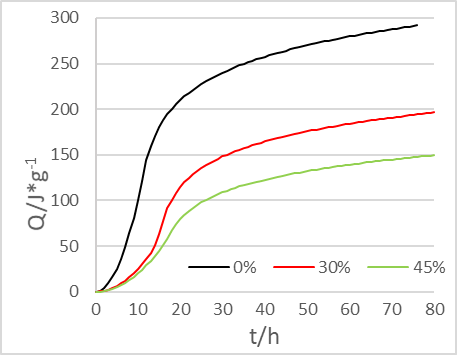Figure 3. Cumulative heat of the composite binding system of copper tailings-cement under different mix ratios

4.2 Micro-morphological differences

To disclose the impact of copper tailings dosage on hydration process, pure slurries of the binding material were performed, and observed under the scanning electron microscope (SEM). From the SEM images, it was learned that the pure cement produced lots of C-S-H gel, Ca(OH)2, and ettringite (AFt) in the early stage, creating a cross-linked continuous mesh (Figure 4(1)). After mixing a certain amount of copper tailings, the hydration products became more diverse, but the integrality of the binding system was weakened (Figure 4(2)). After mixing lots of copper tailings, the structural density decreased, and the amount C-S-H gel was reduced , while more non-gel materials appeared (Figure 4(3)).

## 4.pngFigure 4. SEM images on the composite binding system after 70h hydration

With the growing dosage of copper tailings, the C-S-H gel and AFt contents in the system decreased, compared with those in the system with a lower dosage of copper tailings during the same period. In the meantime, the cement slurry became less dense and looser in structure. Although the strength would increase in the late stage, the overall strength of the binding system eventually decreased. If copper tailings are applied to large volume concrete, this defect could be made up by stepping up grinding and extending calcination, so as to enhance the activity of the materials . Further research is needed to fully overcome this defect.

5. Comparison Between Modeling and Experimental Results

5.1 Calculation results of Krstulovic-Dabic model and experimental results

The model parameters (n, KNG, KI, and KD) after mixing fly ash could be looked up in the literature . Here, the linear relationship between ln[-ln(1-α)] and ln(t-t0) is fitted by Krstulovic-Dabic equation (1), whose slope is the number of reaction phases n, and intercept is nKNG; on this basis, the authors computed the value of KNG . Similarly, the linear relationship between [1-(1-α) 1 /3] and (t-t0) was fitted by formula (3), whose slope is the value of KI (Figure 5(1)); the linear relationship between [1-(1-α) 1 /3]2 and (t-t0) was fitted by formula (5), whose slope is the value of KD (Figure 5(2)). In this way, the hydration kinematics parameters of different dosages of copper tailings were obtained (Table 2).

## 5-1.png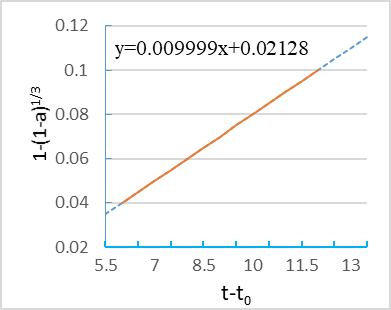(1)

## 5-2.png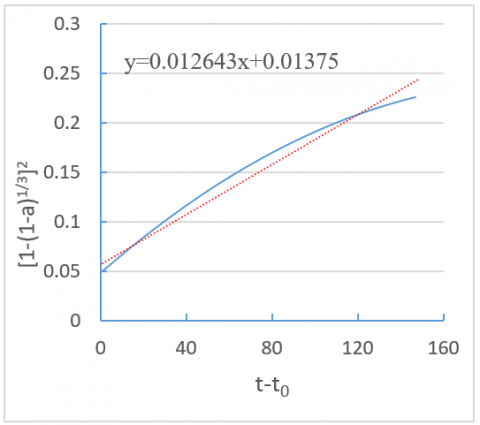(2)

Figure 5. Linearly fitted values of kinematics parameters of copper tailings

Table 2. Hydration kinematics parameters for composite binding material of copper tailings-cement (15℃)

 Dosage n KNG KI KD 0% 2.121 0.0622 0.0149 0.0016 15% 2.014 0.0573 0.0134 0.0017 30% 1.992 0.0512 0.0121 0.0016 45% 1.783 0.0489 0.0108 0.0014

Substituting the values into formulas (2), (4), and (6), the authors obtained the time history of hydration degree. The hydration degree α was converted from the measured heat release by formula (10) (Figure 6).

Next, the hydration rate was characterized by the reduction of hydration particle radius, and the time history of hydration rate was obtained by formulas (11)-(13) (Figure 7).

## 6.pngFigure 6. Calculated and measured values of hydration degree

## 7.png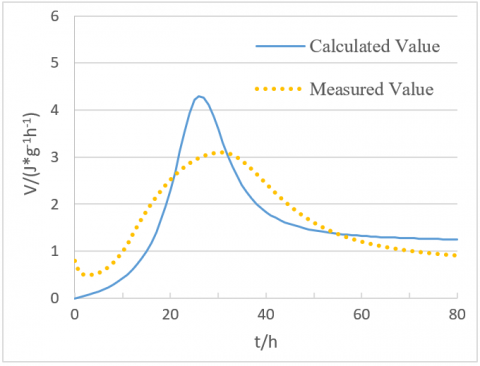Figure 7. Calculated and measured values of hydration rate

Figure 6 compares the calculated and measured values of hydration degree for the composite binding material containing 45% copper tailings at the temperature of 15℃ and water-cement ratio of 0.32. When the hydration degree curve entered the deceleration period, the fitted curve deviated far from the experimental curve. The reasons are as follows: the deceleration period starts when the cement hydration is controlled by diffusion; the hydration products wrap the surface of cement particles, and impedes the hydration inside these particles, thereby hindering the hydration process.

Figure 7 compares the calculated and measured values of hydration rates of the C50 concrete prepared with the composite binding material containing 45% copper tailings, at the water-cement ratio of 0.4. It can be seen that, the high-dose copper tailings widened the gap between the fitted hydration rate curve and the experimental curve, indicating that the admixture does affect cement hydration.

According to the theory of Krstulovic-Dabic model, the binding system with high-dose mineral admixture has a large reaction resistance in Phase NG, which delays the initial setting and slows down the hydration; the slow hydration rate will in turn delay the final setting, and extend the acceleration period. In Phase I, the hydration rate is relatively fast, and the secondary hydration of the mineral admixture begins to dominate the reaction. In Phase D, the hydration rate is still fast, causing an extension of the deceleration period.

The above experimental results indicate that the hydration features of the composite binding material of copper tailings-cement are basically the same as those predicted by the theory of Krstulovic-Dabic model. However, the results calculated by this theory have a large difference from the experimental results.

5.2 Calculation results of center particle hydration model and experimental results

The values of all parameters in Table 3, namely, K1CT20, K2CT20, DeCT20, and krCT20, satisfy the Arrhenius law [25, 26]. According to the Arrhenius equation , the influence coefficients K1CT, K2CT, DCT, and kCT were computed, and substituted into formula (21) to obtain the dominant reaction control coefficient kdCT; the mass of Ca(OH)2 mCH(t) was computed by formula (19). Then, the change law of hydration rate dα/dt with hydration degree α can be obtained by the core formula (18) of our model for the composite binding material of copper tailings-cement at different dosages of copper tailings.

Table 3. Parameters of the hydration model for the composite binding material (20℃)

 w/b Dosage K1CT20 K2CT20 DeCT20 krCT20 Β1CT Β2CT Β3CT ECT/R 0.25 0 0.010 0.382 4.621 6.195 9 804 6000 6000 0.25 30% 0.010 0.279 5.101 6.195 9 804 4500 5000 0.25 45% 0.010 0.218 7.551 6.195 9 804 4300 3000

Figure 8 compares the model results and experimental results on the hydration rate-hydration degree curve at the copper tailings dosage of 30%, water-cement ratio of 0.25, and temperatures of 5℃, 15℃, and 30℃. The experimental data were obtained from Li’s research . The model results agree well with the experimental data, indicating that the proposed hydration kinetics model can accurately simulate the kinetics of the composite binding system of copper tailings-cement in the hydration process, including both acceleration and deceleration periods.

It can be seen from Figure 8 that, with the growing dosage of copper tailings and falling hydration temperature, the hydration exothermic rate of the composite binding system continued to drop; a relatively low construction temperature could effectively suppress the heat release, without affecting the hydration degree and strength growth in the late stage.

Figure 9 compares the model results and experimental results on the hydration rate-hydration degree curve at the copper tailings dosage of 45%, water-cement ratio of 0.25, and temperatures of 5℃, 15℃, and 30℃. The experimental data at the dosage of 45% were not provided in Li’s research . Thus, the situation at that dosage is not compared here.

Comparing Figures 8 and 9, the hydration at the dosage of 45% was slower than that at dosage of 30%, both in the early stage and the entire process. From the curve trends, it can be seen that, when the cement was replaced by high-dose copper tailings, the effective water-cement ratio increased due to the sharp decline in cement content; because of the low calcium content of copper tailings, the composite binding material was weakly alkaline, making it difficult to stimulate the hydration activity of copper tailings powder in the early stage of hydration. With the progression of hydration, the cement in the binding material was hydrated to a greater degree. Then, the binding material became more alkaline, which stimulates the pozzolanic reaction of copper tailings powder. As a result, a growing volume of copper tailings powder was activated for hydration. Under different temperatures, the hydration rate eventually reached around 0.8.

## 8.png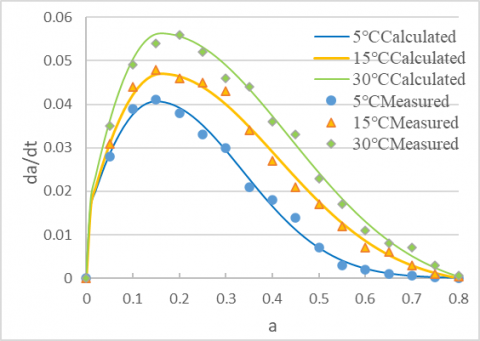Figure 8. Hydration process of the composite binding material with 30% copper tailings at different temperatures

## 9.png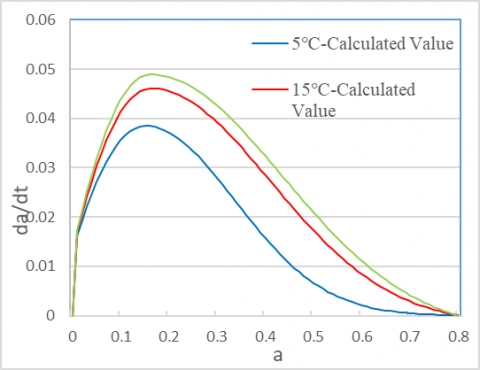Figure 9. Hydration process of the composite binding material with 45% copper tailings at different temperatures

The parameters of the above hydration model were calculated based on the parameters of the fly ash experiment conducted by Chen et al. . The calculated values were highly consistent with the measured values provided by Li . Therefore, our model and formulas are in line with the reality, and the copper tailings processed in this research have similar hydration activity as fly ash.

6. Conclusions

(1) Krstulovic-Dabic model can simualte the hydration process of the composite binding system of low-dose copper tailings-cement. When the dosage is greater than 40%, the hydration features of the composite binding system of high-dose copper tailings-cement can be accurately simulated by the center particle hydration model modified agaisnt the factors affecting copper tailings.

(2) High-dose copper tailings can lower the hydration heat release, slow down hydration rate, improve thermal performance, and reduce cracking probability of the entire hydration process. In the induction period, the mixing of high-dose copper tailings hinders the hydration, and extends the initial and final settings; in the deceleration period, the pozzolanic reaction takes place, giving rise to the third exothermic peak.

(3) The copper tailings powder properly processed under high temperature and grinding is active enough to effectively replace fly ash and GGBS as cement admixtures. When the dosage surpasses 40%, the copper tailings can be applied to mass concrete projects.

Acknowledgment

This work was supported by Provincial Quality Engineering Project of Anhui, China (Grant No.: 2019xfxm71 & 2020-6656).

References

 Li, X., Aruhan, Yan, P.Y. (2010). Research on hydration degree of cement-fly ash complex binders. Journal of Building Materials, 13(5): 584-588. https://doi.org/10.3969/j.issn.1007-9629.2010.05.005

 Han, F.H., Wang, D.M.,Yan, P.Y. (2014). Hydration kinetics of composite binder containing different content of slag or fly ash. Journal of Chinese Ceramic Society, 42(5): 613-620. https://doi.org/10.7521/j.issn.0454-5648.2014.05.10

 Dang, H.F., Long, G.C., Ma, C. (2019). Effect of temperature on hydration kinetics of cement system with mineral admixtures. Journal of Railway Science and Engineering, 16(4): 907-914. https://doi.org/10.19713/j.cnki.43−1423/u.2019.04.010

 Sakata, K.A. (1983). Study on moisture diffusion in drying and drying shrinkage of concrete. Cement and Concrete Research, 13(2): 216-224. https://doi.org/10.1016/0008-8846(83)90104-7.

 Sun, Q., Ding, B., Zheng, Z., Du, R., Qiang, S. (2019). Study on temperature control and crack prevention of mass concrete for large powerhouse constructed in cold area. In MATEC Web of Conferences, 275: 02009. https://doi.org/10.1051/matecconf/201927502009

 Zhang, X.L. (2011). Thermkinetic study on hydration of cementious matirials. Wuhan: Dissertation of Wuhan University of Technology. https://doi.org/10.7666/d.y1880498

 Xie, S.S. (2011). Study on hydration mechanism of cement pozzolanic cementitious system. Wuhan: Dissertation of Changjiang Academy of Sciences.

 Krstulovic, R., Dabic, P. (2000). A conceptual model of the cement hydration process. Cement and Concrete Research. 30(5): 693-698. https://doi.org/10.1016/S0008-8846(00)00231-3

 Li, Q.H., Xiao, L.Z. (2020). Temperature effect of cement-based materials based on hydration kinetics model. Journal of Wuhan Institute of Technology, 42(4): 432-438.

 Wang, P.M., Li, N., Xu, L.L. (2017). Hydration evolution and compressive strength of calcium sulphoaluminate cement constantly cured over the temperature range of 0 to 80℃. Cement and Concrete Research, 100(3): 203-213. https://doi.org/10.1016/j.cemconres.2017.05.025

 Wang, Y.W. (2014). Study of hudration mode of cement with mineral admixtres and its appication. Hangzhou: Dissertation of Zhejiang University.

 Tomosawa, F. (1997). Development of a kinetic model for hydration of cement. Cong Chemistry of Cement, 2(3): 112-119.

 Park, K.B., Jee, N.Y., Yoon, I.S., Lee, H.S. (2008). Prediction of temperature distribution in high-strength concrete using hydration model. ACI Materials Journal, 105(2): 180. https://doi.org/10.1016/j.tsf.2007.04.123

 Escalante, J.I., Gomez, L.Y., Johal, K.K., (2001). Reactivity of blast furnace slag in portland cement blends hydrated under different conditions. Cement and Concrete Research, 31(10): 1403-1409. https://doi.org/10.1016/S0008-8846(01)00587-7

 Saeki, T., Monteiro, P.J. (2005). A model to predict the amount of calcium hydroxide in concrete containing mineral admixtures. Cement and concrete research, 35(10): 1914-1921. https://doi.org/10.1016/j.cemconres.2004.11.018

 Koichi, M., Rajesh, C., Toshiharu, K. (1998). Modeling of concrete performance: hydration, microstructure formation and mass transport. London and New York: Routledge.

 Klemczak, B. (2011). Prediction of coupled heat and moisture transfer in early-age massive concrete structures. Numerical Heat Transfer Part A: Applications, 6(3): 212-233. https://doi.org/10.1080/10407782.2011.594416

 Kundu, S., Aggarwal, A., Mazumdar, S., Dutt, K.B. (2016). Stabilization characteristics of copper mine tailings through its utilization as a partial substitute for cement in concrete: preliminary investigations. Environmental Earth Sciences, 75(3): 1-9. https://doi.org/10.1007/s12665-015-5089-9

 Zhang, J., Wang, J., Gao, Y. (2015). Moisture movement in early-age concrete under cement hydration and environmental drying. Magazine of Concrete Research, 68(78): 391-408. https://doi.org/10.1680/jmacr.15.00293

 Scrivener, K.L., Juilland, P., Monteiro, P.J.M. (2015). Advances in understanding hydration of portland cement. Cement & Concrete Research, 78(12): 38-56. https://doi.org/10.1016/j.cemconres.2015.05.025

 Thomas, B.S., Damare, A., Gupta, R.C. (2013). Strength and durability characteristics of copper tailing concrete. Construction & Building Materials, 48(11): 894-900. https://doi.org/10.1016/j.conbuildmat.2013.07.075

 Esmaeili, J., Aslani, H. (2019). Use of copper mine tailing in concrete: strength characteristics and durability performance. Journal of Material Cycles and Waste Management, 21(3): 729-741. https://doi.org/10.1007/s10163-019-00831-7

 Zhang, Y.S., Sun, W. (2006). Hydration process of Portland cements fly ash pastes. Journal of Southeast University(Natural Science Edition), 36(1): 118-123.

 Chen, J., Shui, Z.H., Sun, T. (2019). Early hydration kinetics research of calcined coal gangue in cement-based materials. Bulletin of the Chinese Ceramic Society, 38(7): 1983-1990.

 Wang, X.Y., Lee, H.S. (2012). Modeling of hydration kinetics in cement based materials considering the effects of curing temperature and applied pressure. Construction & Building Materials, 28(1): 1-13. https://doi.org/10.1016/j.conbuildmat.2011.08.037

 Chen, W., Brouwers, H.J.H. (2008). Mitigating the effects of system resolution on computer simulation of Portland cement hydration. Cement and Concrete Composites, 30(9): 779-787. https://doi.org/10.1016/j.cemconcomp.2008.06.001

 Lam, L., Wong, Y.L., Poon, C.S. (2000). Degree of hydrat ion and gel / space ratio of high-volume fly ash / cement systems. Cement and Concrete Research, 30(5): 747-756. https://doi.org/10.1016/S0008-8846(00)00213-1

 Li, Q.L. (2018). Mechanism effects of copper tailings in cement-based materials. Wuhan: Dissertation of Wuhan University.